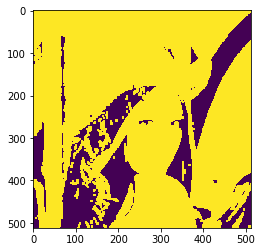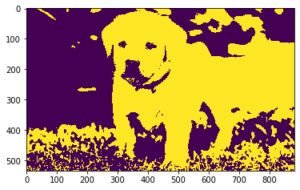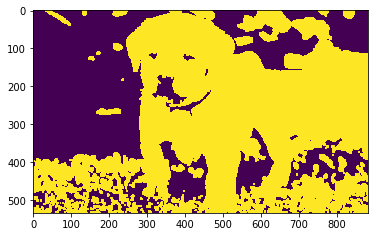Mahotas – Convolution of Image

• Last Updated : 29 Jul, 2021

In this article, we will see how we can do convolution of the image in mahotas. Convolution is a simple mathematical operation which is fundamental to many common image processing operators. Convolution provides a way of `multiplying together’ two arrays of numbers, generally of different sizes, but of the same dimensionality, to produce a third array of numbers of the same dimensionality.

In this tutorial, we will use “lena” image, below is the command to load it.

Below is the lena imageIn order to do this we will use mahotas.convolve method

Syntax : mahotas.convolve(img, weight)

Argument : It takes image object and numpy nd array objectas argument

Return : It returns image object

Note : Input image should be filtered or should be loaded as grey

In order to filter the image we will take the image object which is numpy.ndarray and filter it with the help of indexing, below is the command to do this

image = image[:, :, 0]

Below is the implementation

Python3

 # importing required librariesimport mahotasimport mahotas.demosfrom pylab import gray, imshow, showimport numpy as np   # loading imageimg = mahotas.demos.load('lena')   # filtering imageimg = img.max(2) # otsu methodT_otsu = mahotas.otsu(img)     # image values should be greater than otsu valueimg = img > T_otsu   print("Image threshold using Otsu Method")   # showing imageimshow(img)show()   # weightweight = np.ones((5, 5), float) # convolving imagenew_img = mahotas.convolve(img, weight)  print("Convolved Image") # showing imageimshow(new_img)show()

Output :

Image threshold using Otsu MethodConvolved ImageAnother example

Python3

 # importing required librariesimport mahotasimport numpy as npfrom pylab import gray, imshow, showimport os  # loading imageimg = mahotas.imread('dog_image.png')  # filtering imageimg = img[:, :, 0]   # otsu methodT_otsu = mahotas.otsu(img)     # image values should be greater than otsu valueimg = img > T_otsu   print("Image threshold using Otsu Method")   # showing imageimshow(img)show()   # weightweight = np.ones((5, 5), float) # convolving imagenew_img = mahotas.convolve(img, weight)  print("Convolved Image") # showing imageimshow(new_img)show()

Output :

Image threshold using Otsu MethodConvolved ImageAttention geek! Strengthen your foundations with the Python Programming Foundation Course and learn the basics.

To begin with, your interview preparations Enhance your Data Structures concepts with the Python DS Course. And to begin with your Machine Learning Journey, join the Machine Learning - Basic Level Course

My Personal Notes arrow_drop_up